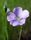## SAS Optimization Model

Hi, I am trying to write a code for a transshipment procurement model from 4 plants (Ps) via 3 transshipment nodes (Ts) to 4 destination distribution centers (DCs).

The conditon is that each node in the inflow (from Ps to Ts) and outflow (from Ts to Ds) variables need to be a multiple of 3.

I have tried to do that via introducing the "lot" variable, however it doesn't work correctly when I use lot =3, but it does give a correct answer when lot size = 1.

Can someone kindly, help point out the mistake I am making. Thank you.

My Current Code -

``````proc optmodel;
set Ps ={'P1', 'P2', 'P3', 'P4'};
set Ds ={'D1', 'D2', 'D3', 'D4'};
set Ts ={'T1', 'T2', 'T3'};
number InCost{Ps, Ts} = [
39	29	43
40	35	43
48	44	31
37	39	30];
number OutCost{Ts, Ds} = [
34	17	36	25
30	47	16	15
29	22	21	23];
number Supply{Ps} = [37	34	20	28];
number Demand{Ds} = [18	15	21	3];
number TransshipmentCapacity {Ts} = [37	37	43];
number TransshipmentFixedCost{Ts} = [700	700	900];
number ProductionFixedCost{Ps} = [420	120	240	580];
var inflow {Ps, Ts} integer>=0;
var outflow{Ts, Ds} integer>=0;
number lot = 1;
var y{Ts} binary;
var a{Ps} binary;
number M=1000000;
number N=1000000;
minimize Z = sum{i in Ps}sum{j in Ts}(inflow[i,j]*lot)*InCost[i,j]+sum{j in Ts}y[j]*TransshipmentFixedCost[j]
+sum{i in Ps}a[i]*ProductionFixedCost[i]+sum{j in Ts}sum{k in Ds}(outflow[j,k]*lot)*OutCost[j,k];
con SupplyConst {i in Ps}:sum{j in Ts}(inflow[i,j]*lot)  <= Supply[i];
con DemandConst {k in Ds}:sum{j in Ts}(outflow[j,k]*lot) >= Demand[k];
con NoStockConst{j in Ts}:sum{i in Ps}(inflow[i,j]*lot) = sum{k in Ds}(outflow[j,k]*lot);
con TsCapConst  {j in Ts}:sum{i in Ps}(inflow[i,j]*lot) <= TransshipmentCapacity[j];
con linkingy {j in Ts}:sum{i in Ps}(inflow[i,j]*lot)<=M*y[j];
con linkinga {i in Ps}:sum{j in Ts}(inflow[i,j]*lot)<=N*a[i];
solve;
print inflow;
print outflow;
print y;
print a;
print Z;
print {j in Ts} (sum{i in Ps}inflow[i,j]);
print {j in Ts} (sum{k in Ds}outflow[j,k]);
quit;``````
4 REPLIES 4Ksharp
Super User

## Re: SAS Optimization Model

Please post it at OR forum .

## Re: SAS Optimization ModelLinusH
Tourmaline | Level 20

## Re: SAS Optimization Model

@Ksharp: as Super User, you have the power to move it there 🙂
Data never sleeps

## Re: SAS Optimization Model

Discussion stats
• 4 replies
• 998 views
• 1 like
• 4 in conversation